Technical Article

# Multiplication Examples Using the Fixed-Point Representation

December 15, 2017 by Dr. Steve Arar

## This article will discuss several multiplication examples using the fixed-point representation.

To perform fixed-point multiplication, we can first ignore the binary point of the multiplier and multiplicand, perform the multiplication treating the operands as two’s complement numbers, and, then, determine the position of the binary point for the result.

The pencil-and-paper method of binary multiplication is just like the pencil-and-paper method of decimal multiplication. There are two phases: first, the partial products are generated, and, then, these partial products are added together to obtain the final result. This is, in fact, based on the idea that multiplication is the serial addition of one number to itself. Example 1 below elaborates this procedure. Then, we will discuss the cases where we need to deal with signed numbers.

### Unsigned by Unsigned Multiplication

Example 1: Assume that $$a=101.001_2$$ and $$b=100.010_2$$ are two unsigned numbers in Q3.3 format (to read about the Q-format representation please see this article). Find the product of $$a \times b$$.

Considering the position of the binary point, we can represent $$a$$ and $$b$$ as

$$a=\big(101001 \big)_{2} \times \big (2^{-3} \big)_{10}$$

$$b=\big(100010 \big)_{2} \times \big (2^{-3} \big)_{10}$$

Then, we can write $$a \times b$$ as

$$a \times b= \bigg( \big(101001 \big)_{2} \times \big (2^{-3} \big)_{10} \bigg) \times \bigg( \big(100010 \big)_{2} \times \big (2^{-3} \big)_{10} \bigg)$$

which simplifies to

$$a \times b= \bigg( \big(101001 \big)_{2} \times \big(100010 \big)_{2} \bigg) \times \big( 2^{-6} \big)_{10}$$

This means that we can ignore the binary point of $$a$$ and $$b$$, perform the multiplication, and, then, put the binary point to the left of the sixth bit of the product to obtain the correct multiplication result.

Hence, here, we will first neglect the binary points of $$a$$ and $$b$$. Now, to perform the multiplication, we must sequentially pick one digit of the multiplier from right to left, find the product of this digit by the multiplicand to obtain the corresponding partial product. Then, in a manner similar to the decimal multiplication, we should appropriately shift the partial products to the left and add them together.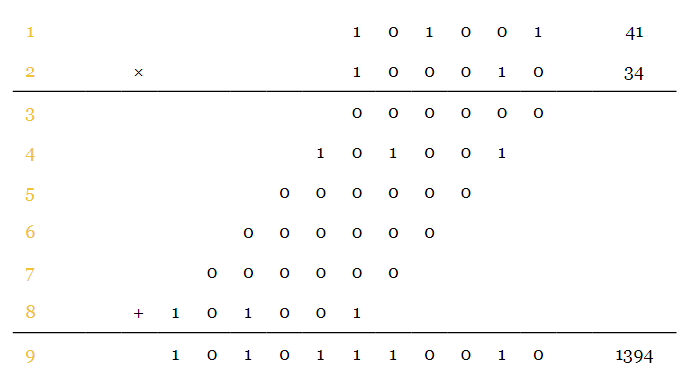The decimal equivalent values of the multiplicand, multiplier, and the result are given to verify the binary multiplication. The numbers in orange show the line number for the future reference. Now, we should put the binary point to the left of the sixth bit of the product which gives $$a \times b=10101.110010_2=21.78125_{10}$$.

The product can be much larger than the multiplier and multiplicand. Hence, we need a sufficiently long wordlength for the product to represent the result correctly. How many bits do we need for the product to prevent any potential overflow? Consider the sum of the first two partial products above (sum of lines 3 and 4). In this case, we are adding a six-bit number $$p_1=000000_2$$ to a seven-bit number $$p_2=1010010_2$$ (note that the second partial product is shifted to the left by one bit). The sum of these two numbers, $$p_1+p_2$$, can be an eight-bit number in general (one bit longer than $$p_2$$). Let’s denote the third partial product shifted to the left by two bits by $$p_3$$, i.e. $$p_3=00000000$$. Adding $$p_3$$ to $$p_1+p_2$$, we are adding two eight-bit numbers and, in general, the result can be a nine-bit number (one bit longer than $$p_3$$). Continuing this procedure, we observe that the product can be one bit longer than the appropriately shifted version of the last partial product. For example, the last partial product for the above multiplication is shifted by five bits. Hence, we have $$p_6=10100100000_2$$ which is an 11-bit number, as a result, the product, $$a \times b$$, can take at most $$12$$ bits. Considering the general form of this example, you can easily calculate the required wordlength for the product of two numbers. In general, if $$a$$ and $$b$$ are two numbers of length $$L_a$$ and $$L_b$$ bits, respectively, we will have to use $$L_a+L_b$$ bits to avoid overflow when representing $$a \times b$$.

The results of Example 1 can be summarized as follows:

Assume that $$a$$ and $$b$$ are two numbers in $$Qn1.m1$$ and $$Qn2.m2$$ formats, respectively. The product $$a \times b$$ will be in $$Qn.m$$ format where $$n=n1+n2$$ and $$m=m1+m2$$. This product wordlength, i.e. $$n+m$$, is long enough to avoid any potential overflow. To find $$a \times b$$, we can ignore the binary point of $$a$$ and $$b$$, perform the multiplication, and, then, put the binary point to the left of the $$m$$th bit of the product to obtain the correct multiplication result.

### Signed by Unsigned Multiplication

Example 2: Assume that $$a=101.001_2$$ and $$b=100.010_2$$ are two numbers in Q3.3 format. Assume that $$a$$ is a signed number but $$b$$ is unsigned. Find the product of $$a \times b$$.

Here the numbers are the same as the first example; however, in this example, $$a$$ is considered to be a signed number. What difference does this make? The partial products of the previous example were unsigned. For example, the second partial product (line 4) was obtained by multiplying $$1_2$$ by a positive number, i.e. $$101001_2$$. However, in this example, the multiplicand $$a$$ is signed and, hence, all of the partial products will be signed numbers. In a previous article, we discussed that, when adding two signed numbers of different lengths, we need to perform sign extension otherwise the result can be invalid. Hence, to perform a signed-by-unsigned multiplication, we have to sign-extend the partial products. We first ignore the binary points and obtain: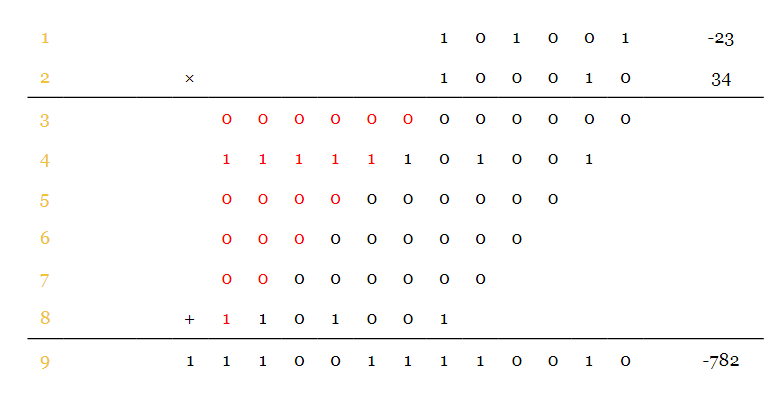Comparing this example with the previous one, you can see that the only difference is in sign-extending the partial products (the bits from sign extension are shown in red). Note that, since we are multiplying two six-bit numbers, in general, the result will be of length $$12$$. Hence, all of the partial products are sign-extended by 12 bits (see this article). Moreover, since the signed numbers are using the two’s complement representation, we know that the addition is performed in modulo-M arithmetic where $$M=2^{12}$$ for this particular example (see this article). Hence, we should discard the 13th bit of the above calculations. Considering the position of the binary point, we obtain

$$a \times b=110011.110010_2=- ( 001100.001110_2) =-12.21875_{10}$$. This is consistent with the result obtained from the equivalent decimal values above, i.e. $$-12.21875=-\tfrac{782}{64}$$.

Example 3: Assume that $$a=100.000_2$$ and $$b=111.111_2$$ are two numbers in Q3.3 format. Assume that $$a$$ is a signed number but $$b$$ is unsigned. Find the product of $$a \times b$$.

This is similar to the previous example. The multiplication can be performed as shown below: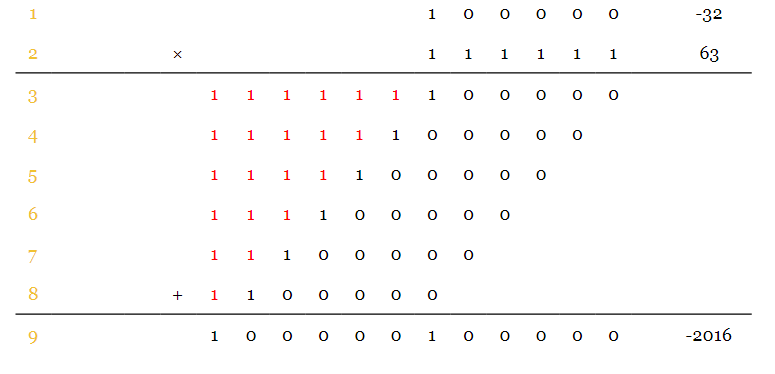To make the calculations easier, you can add the partial products two by two. After each addition, you can discard the bit to the left of the sign bit. Taking the position of the binary point into account, we obtain $$a \times b=100000.100000_2$$.

Before continuing our discussion, we need to review an important feature of the two’s complement representation.

### An Important Feature of the Two’s Complement Representation

Assume that $$x= \big (x_{M-1}x_{M-2} \dots x_0 \big )_2$$ is a binary number in two’s complement format. Then, we have

$$x=-x_{M-1} \times 2^{M-1}+ \sum_{i=0}^{M-2}x_{i} \times 2^{i}$$

##### Equation 1

This means that we can find the equivalent decimal value of a two’s complement number in the same way we convert an unsigned number from binary to the decimal representation except that we must interpret the sign bit with a negative weight. As an example, assume that $$x=101_2$$ is a number in the two’s complement format. Using Equation 1, we have

$$x=-1 \times 2^{2} + 0 \times 2^{1} + 1 \times 2^{0} = -3$$

##### Equation 2

We can obtain the same result with the more common way of finding the equivalent decimal value of a negative two’s complement number, i.e. since $$x$$ is negative we have $$x=-(011_2)=-3_{10}$$.

The proof of Equation 1 is simple. If the sign bit is zero, Equation 1 is obviously valid. Assume that $$x$$ is a negative number i.e. $$x_{M-1}=1$$. Since $$x$$ is an $$M$$-bit number, its equivalent decimal value is obtained by $$-(two's \; complement \; of \; x)=-(2^M-x)$$ (see this article). In the following equations, we will, respectively, use the subscript “Two’s Complement” and “Two” to clarify where we are interpreting $$x$$ as a two’s complement number and where we are interpreting it as an unsigned number. Considering $$x_{M-1}=1$$, we have

\begin{align} \bigg( 1 \; x_{M-2}...x_{0} \bigg)_{Two's \; Complement} &=- \bigg( 2^M - \big( 1 \; x_{M-2}...x_{0} \big)_{Two} \bigg) \\ &=- 2^M + 2^{M-1} + \sum_{i=0}^{M-2}x_{i} \times 2^{i} \\ &= - 2^{M-1} + \sum_{i=0}^{M-2}x_{i} \times 2^{i} \end{align}

##### Equation 3

Now, we can continue our discussion of multiplication in the fixed-point representation.

### Unsigned by Signed Multiplication

Example 4: Assume that $$a=01.001_2$$ and $$b=10.010_2$$ are two numbers in Q2.3 format. Assume that $$a$$ is an unsigned number but $$b$$ is signed. Find the product of $$a \times b$$.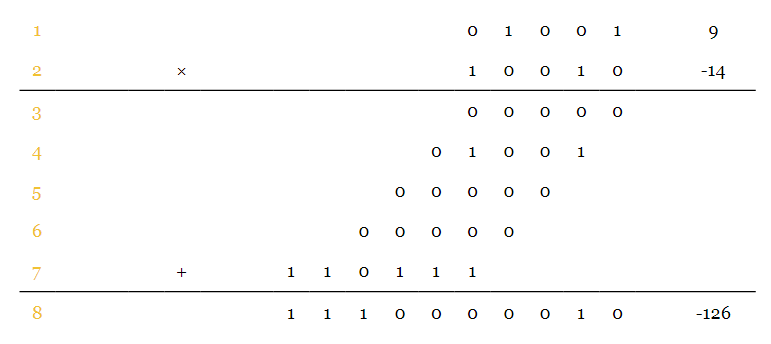Please pay attention to the last partial product (line 7). Since the multiplier, $$b$$, is a signed number, its most significant bit (MSB) is the sign bit. As proved by Equation 3, the equivalent decimal value of a two’s complement number can be found by treating the number as an unsigned number except that we must interpret the sign bit with a negative weight. Due to this property of two’s complement numbers, we can perform the above multiplication treating the multiplier as an unsigned number; however, we will have to consider a negative weight for the MSB. Hence, as shown in the calculations of this example, the last partial product is obtained by calculating the two’s complement of $$a$$. Considering the position of the binary point, we obtain $$a \times b=1110.000010_2$$. The result is obviously a signed number because our last partial product was signed. To find the equivalent decimal value of this negative number, we can write $$a \times b=-(0001.111110_2)=-1.96875_10$$. This is consistent with the decimal calculations, i.e. $$-1.96875=-\tfrac{126}{64}$$.

Here, we need to emphasize on two points: first, since all of the partial products except the last one are unsigned, we only need to sign-extend the last partial product. Second, when calculating the two’s complement corresponding to the last partial product, we must represent the multiplicand with one bit longer. For example, in the above calculations, we are considering $$a$$ to be $$001001$$, and, then, find its two’s complement. This gives us the last partial product as $$110111$$. The wrong alternative is to first find the two’s complement of the five-bit $$a$$, which gives $$10111$$, and then sign-extend the result which leads to $$110111$$. Although, in this particular example, these two calculations give the same result, this is not the case in general. The next example, further clarifies this point.

Example 5: Assume that $$a=11.001_2$$ and $$b=10.010_2$$ are two numbers in Q2.3 format. Assume that $$a$$ is an unsigned number but $$b$$ is signed. Find the product of $$a \times b$$.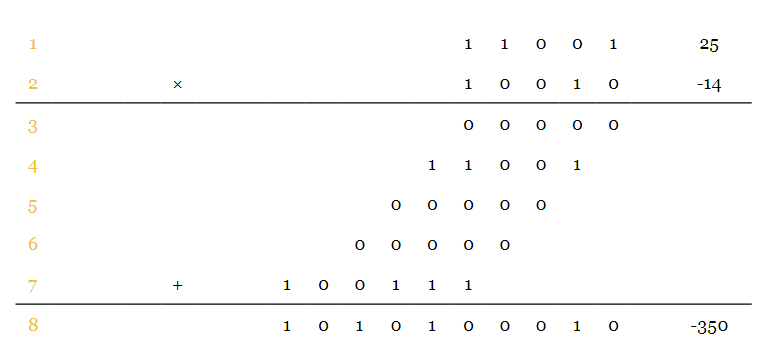Considering the position of the binary point, we obtain $$a \times b=1010.100010_2$$. The result is obviously a signed number because our last partial product was signed. Hence, we have $$a \times b=-(0101.011110_2)=-5.46875_{10}$$. This is consistent with the decimal calculations, i.e. $$-5.46875=-\tfrac{350}{64}$$.

When calculating the two’s complement corresponding to the last partial product, we must represent the multiplicand with one bit longer. For example, in the above calculations, we are considering $$a$$ to be $$011001$$, and, then, find its two’s complement. This gives us the last partial product as $$100111$$. This signed partial product is negative. We were expecting this number to be negative because $$a$$ is unsigned but the MSB of $$b$$ has a negative weight. Now, let’s examine the wrong alternative. This time, we first find the two’s complement of the five-bit a, which gives $$00111$$, and then sign extending the result which leads to $$000111$$. These calculations give us a positive number which is not correct. To summarize, when we are calculating the two’s complement corresponding to the last partial product, we must represent the multiplicand with one bit longer and, then, find its two’s complement.

### Signed by Signed Multiplication

Example 6: Assume that $$a=11.001_2$$ and $$b=10.010_2$$ are two signed numbers in Q2.3 format. Find the product of $$a \times b$$.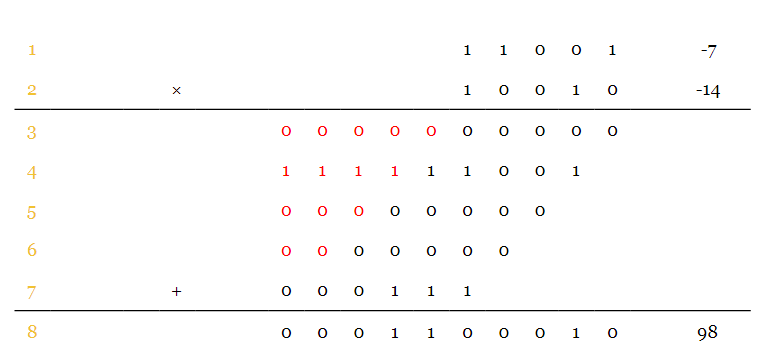Similar to the signed-by-unsigned multiplication, the partial products are signed and, to perform the addition correctly, we need to sign-extend the partial products (except the last partial product which will be discussed in a minute). Since we are multiplying two five-bit numbers, in general, the result will be of length $$10$$. Hence, the partial products are sign-extended by $$10$$ bits.

Similar to the unsigned-by-signed multiplication, we have to consider a negative weight for the MSB of $$b$$. Hence, as shown in the above calculations, the last partial product is obtained by calculating the two’s complement of $$a$$. When calculating this two’s complement, we must represent the multiplicand, $$a$$, with one bit longer and, then, find its two’s complement. Since here $$a$$ is a signed number, representing it with one bit longer is equal to sign-extending it by one bit. The next example clarifies how one may take a wrong approach when calculating this last partial product.

Example 7: Assume that $$a=10.000_2$$ and $$b=10.010_2$$ are two signed numbers in Q2.3 format. Find the product of $$a \times b$$.

Based on the above example, we have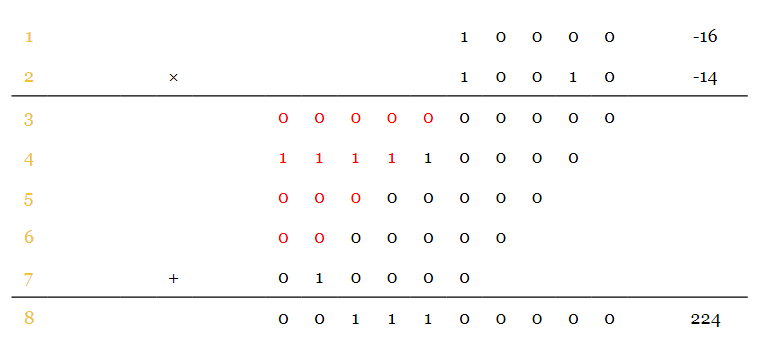Considering the decimal equivalents, we observe that the calculations are valid. Now, let’s examine a potential mistake when calculating the above multiplication. In this case, when calculating the last partial product, we first find the two’s complement of $$a$$ and then sign-extend it. Hence, we have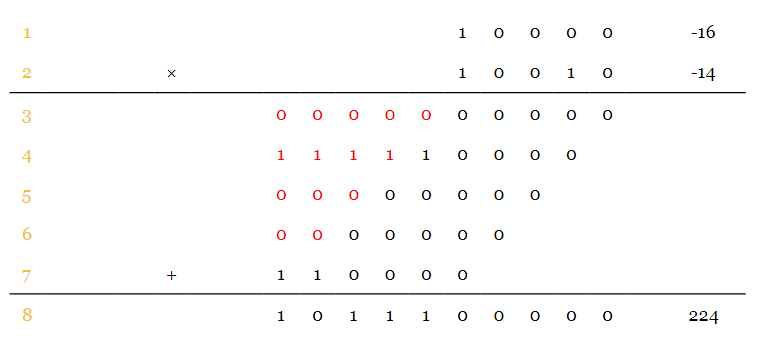We observe that the result is a negative number while the decimal calculations lead to a positive value. Hence, in general, we should first sign-extend $$a$$ and then find its two’s complement.

### Summary

• Assume that $$a$$ and $$b$$ are two numbers in Qn1.m1 and Qn2.m2 formats, respectively. The product $$a \times b$$ will be in Qn.m format where n=n1+n2 and m=m1+m2. This product wordlength, i.e. n+m, is long enough to avoid any potential overflow. To find $$a \times b$$, we can ignore the binary point of $$a$$ and $$b$$, perform the multiplication, and, then, put the binary point to the left of the $$m$$th bit of the product to obtain the correct multiplication result.
• We can find the equivalent decimal value of a two’s complement number in the same way we convert an unsigned number from binary to the decimal representation except that we must interpret the sign bit with a negative weight.
• For the unsigned-by-signed and signed-by-signed multiplications, we have to consider a negative weight for the MSB of the multiplier. As a result, the last partial product is obtained by calculating the two’s complement of the multiplicand. When calculating this two’s complement, we must represent the multiplicand with one bit longer and, then, find its two’s complement.
• When the partial products are signed, we need to sign-extend them to perform the additions correctly.

1 Comment•ali69550 August 13, 2020

Hi,
I think that in the following sentence in the summary part:
“Assume that a and b are two numbers in Qn1.m1 and Qn2.m2 formats, respectively. The product a×b will be in Qn.m format where n=n1+n2 and m=m1+m2.”
we’d better say that it holds for unsigned numbers, because you defined Qn.m format as n including the sign bit.
thanks,
regards
Ali G. Dezfuli

Like.